# Central Force Motions (Part - 1)- Classical Mechanics, UGC - NET Physics Physics Notes | EduRev

## Physics : Central Force Motions (Part - 1)- Classical Mechanics, UGC - NET Physics Physics Notes | EduRev

The document Central Force Motions (Part - 1)- Classical Mechanics, UGC - NET Physics Physics Notes | EduRev is a part of the Physics Course Physics for IIT JAM, UGC - NET, CSIR NET.
All you need of Physics at this link: Physics

Reduction to the Equivalent One-body Problem – the Reduced Mass

We consider a system consisting of two point masses, m1 and m2 , when the only forces are those due to an interaction potential U . We will assume that U is a function of the distance between the two particles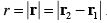Such a system has six degrees of freedom. We could choose, for example, the three components of each of the two vectors, r1 and r(see Figure 6-1). However, since the potential energy is solely a function of the distance between the two particles, i.e., U = U ( r ) , it is to our advantage if we also express the kinetic energy as function of r (that is, of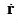). Let’s first define the center of mass R of the system as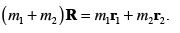(6.1)

We also consider the distance between each particle and the center of mass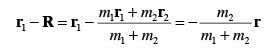(6.2)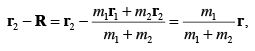with r = r2 - r1 .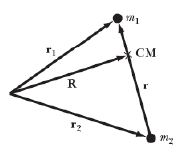Figure 6-1 – The different vectors involved in the two-body problem.

We now calculate the kinetic energy of the system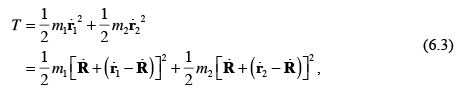and inserting equations (6.2) in equation (6.3) we get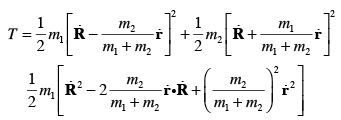(6.4)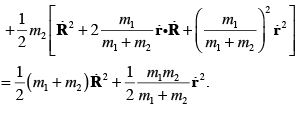We introduce a new quantity µ , the reduced mass, defined as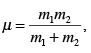(6.5)

which can alternatively be written as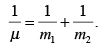(6.6)

We can use equation (6.5) to write the kinetic energy as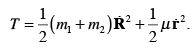(6.7)
We have, therefore, succeeded in expressing the kinetic energy as a function of. We are now in a position to write down the Lagrangian for the central force problem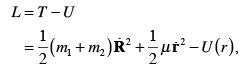(6.8)

where the potential energy U ( r ) is yet undefined, except for the fact that it is solely a function of the distance between the two particles. It is, however, seen from equation (6.8) that the three components of the center of mass vector are cyclic. More precisely,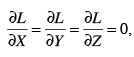(6.9)

with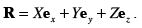The center of mass is, therefore, either at rest or moving uniformly since the equations of motion for X , Y , and Z can be combined into the following vector relation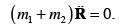(6.10)

Since the center of mass vector (and its derivative) does not appear anywhere else in the Lagrangian, we can drop the first term of the right hand side of equation (6.8) in all subsequent analysis and only consider the remaining three degrees of freedom. The new Lagrangian is therefore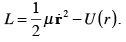(6.11)

What is left of the Lagrangian is exactly what would be expected if we were dealing with a problem of a single particle of mass µ subjected to a fixed central force. Thus, the central force motion of two particles about their common center of mass is reducible to an equivalent one-body problem.

The First Integrals of Motion

Since we are dealing with a problem where the force involved is conservative, where the potential is a function of the distance r of the reduced mass to the force center alone, the system has spherical symmetry. From  Noether’s theorem,  we know that for such systems the angular momentum is conserved . That is,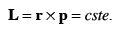(6.12)

From the definition of the angular momentum itself we know that L is always parallel to vectors normal to the plane containing r and p . Furthermore, since in this case L is fixed, it follows that the motion is at all time confined to the aforementioned plane. We are, therefore, fully justified to use polar coordinates as the two remaining generalized coordinates for this problem (i.e., we can set the third generalized coordinate, say, z to be a constant since the motion is restricted to a plane). Since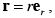we have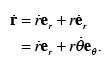(6.13)

In the last equation we have used the following relation (with the usual transformation between the ( r,θ ) polar and the ( x, y ) Cartesian coordinates)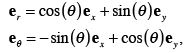(6.14)

from which it can be verified that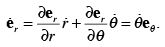(6.15)

We can now rewrite the Lagrangian as a function of r and θ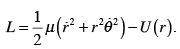(6.16)

We notice from equation (6.16) that θ is a cyclic variable. The corresponding generalized momentum is therefore conserved, that is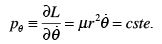(6.17)

The momentum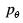is a first integral of motion and is seen to equal the magnitude of the angular momentum vector. It is customarily written as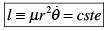(6.18)

As the particle (i.e., the reduced mass) moves along its trajectory through an infinitesimal angular displacement dθ within an amount of time dt , the area dA swept out by its radius vector r is given by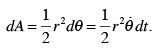(6.19)

Alternatively, we can define the areal velocity as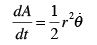(6.20)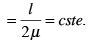Thus, the areal velocity is constant in time. This result, discovered by Kepler for planetary motion, is called Kepler’s Second Law. It is important to realize that the conservation of the areal velocity is a general property of central force motion and is not restricted to the inverse-square law force involved in planetary motion.

Another first integral of motion (the only one remaining) concerns the conservation of energy. The conservation is insured because we are considering conservative systems.
Writing E for the energy we have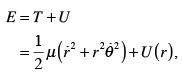(6.21)

or using equation (6.18)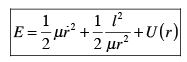(6.22)

The Equations of Motion

We will use two different ways for the derivation of the equations of motion. The first one consists of inverting equation (6.22) and express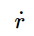as a function of E, l, and U ( r ) such that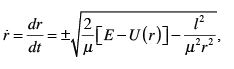(6.23)

or alternatively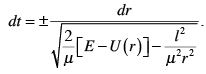(6.24)

Equation (6.24) can be solved, once the potential energy U ( r ) is defined, to yield the solution t = t ( r ) , or after inversion r = r (t ) . We are, however, also interested in determining the shape of the path (or orbit) taken by the particle. That is, we would like to evaluate r = r (θ) or θ = θ ( r ) . To do so we use the following relation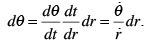(6.25)

Inserting equations (6.18) and (6.23) into equation (6.25), we get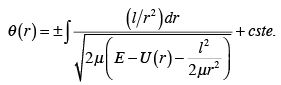(6.26)

It is important to note that the integral given by equation (6.26) can be solved analytically only for certain forms of potential energy. Most importantly, if the potential energy is of the form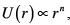for n = 2, - 1, and - 2 the solution is expressible in terms of circular functions.

The second method considered here for solving the equations of motion uses the Lagrange equations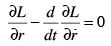(6.27)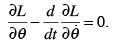The second of these equations was already used to get equation (6.18) for the conservation of angular momentum. Applying the first of equations (6.27) to the Lagrangian (equation (6.16)) gives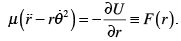(6.28)

We now modify this equation by making the following change of variable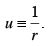(6.29)

We calculate the first two derivatives of u relative to θ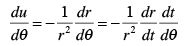(6.30)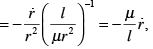where we have used the fact that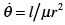, and for the second derivative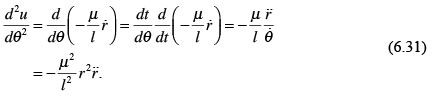From this equation we have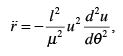(6.32)

and from equation (6.18)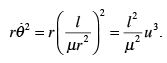(6.33)

Inserting equations (6.32) and (6.33) in equation (6.28) yields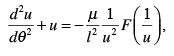(6.34)

which can be rewritten as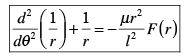(6.35)

Equation (6.35) can be used to find the force law that corresponds to a known orbit r = r (θ) .

Examples

1. Let’s consider the case where the orbit is circular, i.e., r = cste . Then from equation (6.35) we find that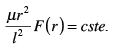(6.36)

Equation (6.36) implies that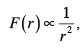(6.37)

which is the functional form of the gravitational force.

2. Let’s assume that we have an orbit given by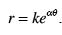(6.38)

From the second derivative of equation (6.38) relative to θ we have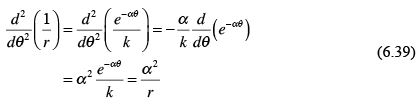and using equation (6.35), we get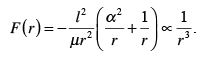(6.40)

Offer running on EduRev: Apply code STAYHOME200 to get INR 200 off on our premium plan EduRev Infinity!

159 docs

,

,

,

,

,

,

,

,

,

,

,

,

,

,

,

,

,

,

,

,

,

,

,

,

;